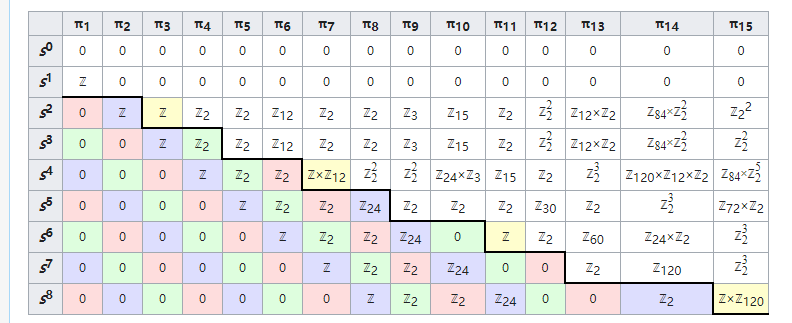# 代数拓扑简介（上）：同伦论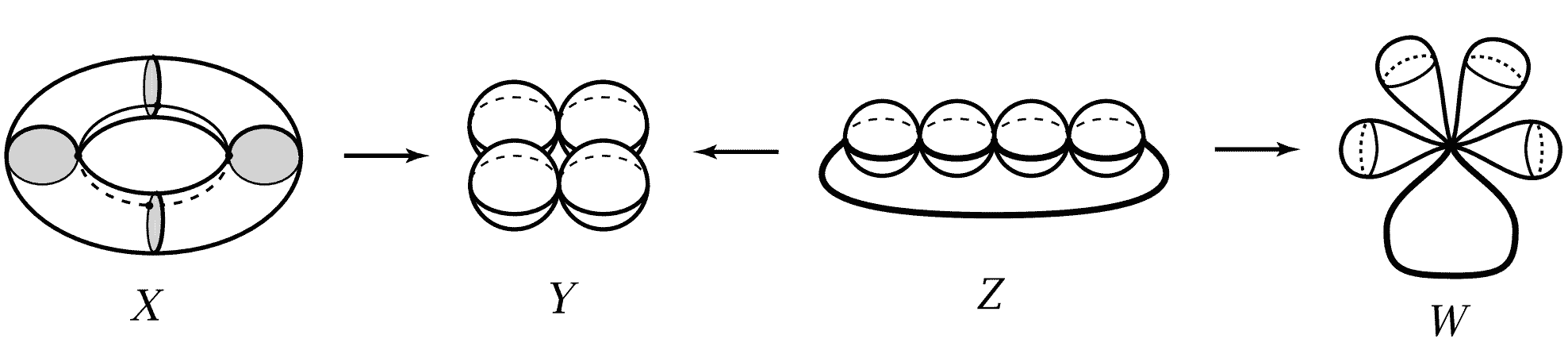## 拓扑学简介

1. 把圆对应到断开的圆时，开口处有跳跃，这种不连续性是拓扑学不允许的，因此圆周与圆弧不同胚；2. 字母O与字母Q的形状不同胚，因为Q的尾巴如果映射到O上一点，则O上那一个点对应Q尾巴上的所有点，不满足一一对应关系，如果Q的尾巴映射到O上的一小段弧，那它跟Q的圈重合了，也不满足一一映射要求。
3. 任意开区间$(a,b)$与直线(可理解为实数轴$\mathbb{R}$)同胚，因为函数$f(x)=tan(x)$把区间$(-\pi/2,\pi/2)$中的点一一映射到$(-\infty,\infty)$，而反正切函数值也是唯一的，满足一一对应条件。对于其它任意开区间$(a,b)$显然能够通过缩放平移到区间$(-\pi/2,\pi/2)$。注意，闭区间$[a,b]$不与直线同胚，因为闭区间包含端点$a$与$b$，它们在直线上找不到对应的点。
4. 平面与挖掉一点的球面同胚。我们可以通过球极投影映射构造这个同胚：除了北极点外，球面上任何一点都能唯一地投影到南极点的切平面上。

## 路径同伦与同伦基本群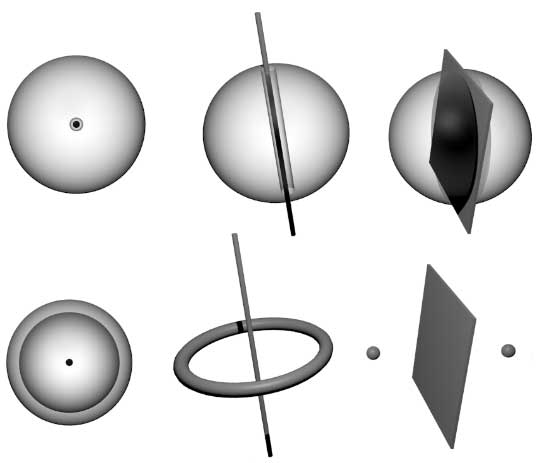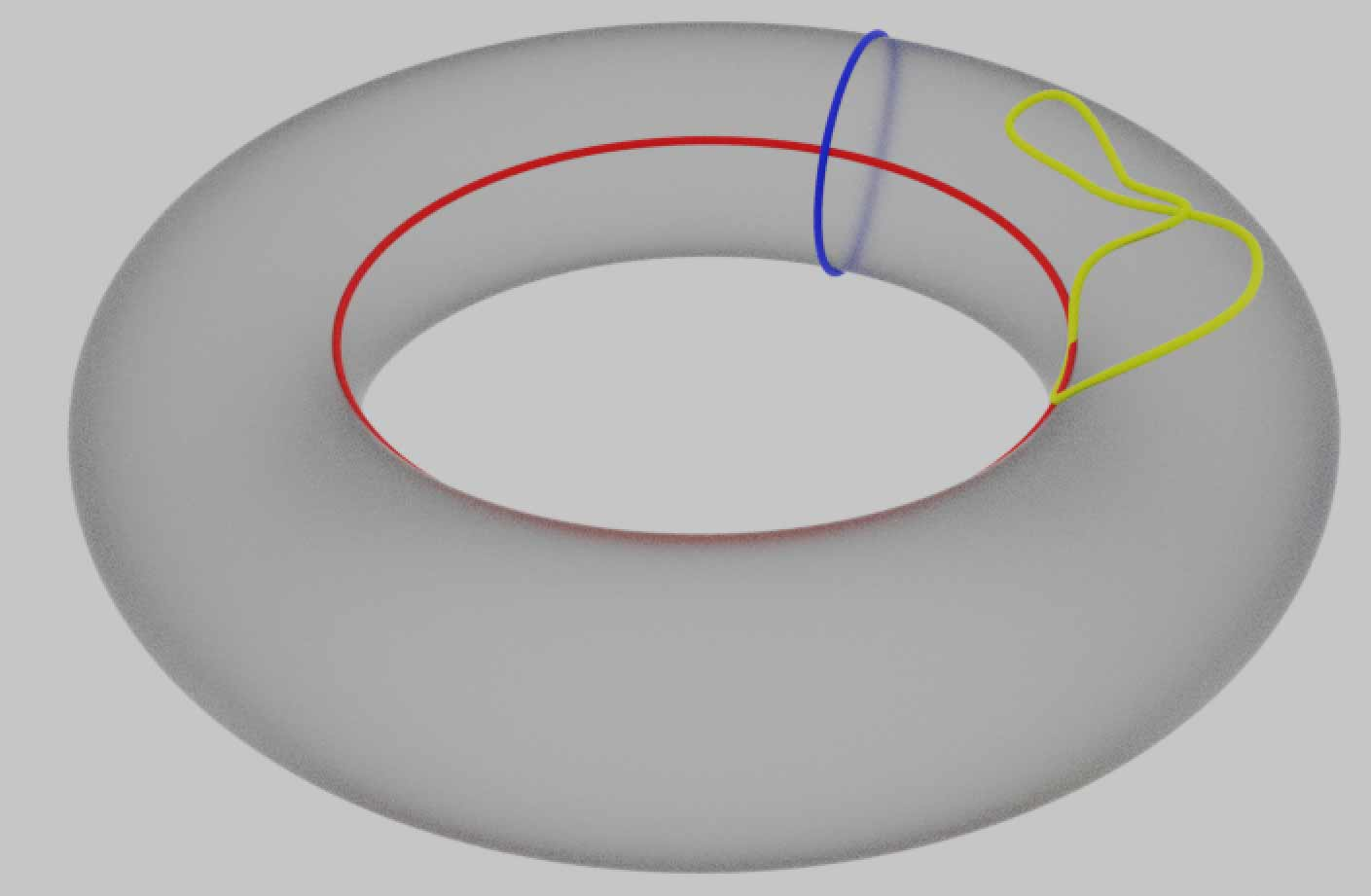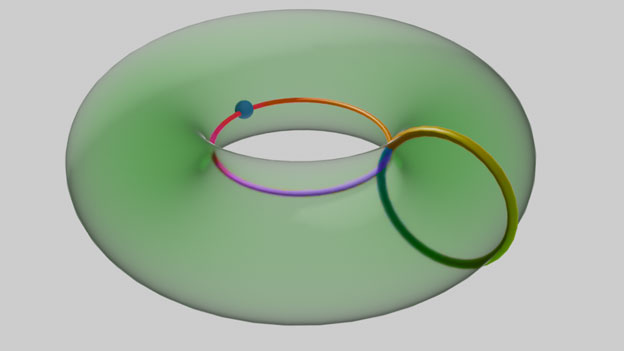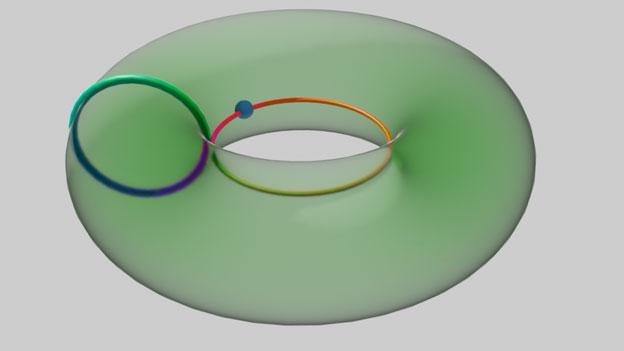## 拓扑空间之间的同伦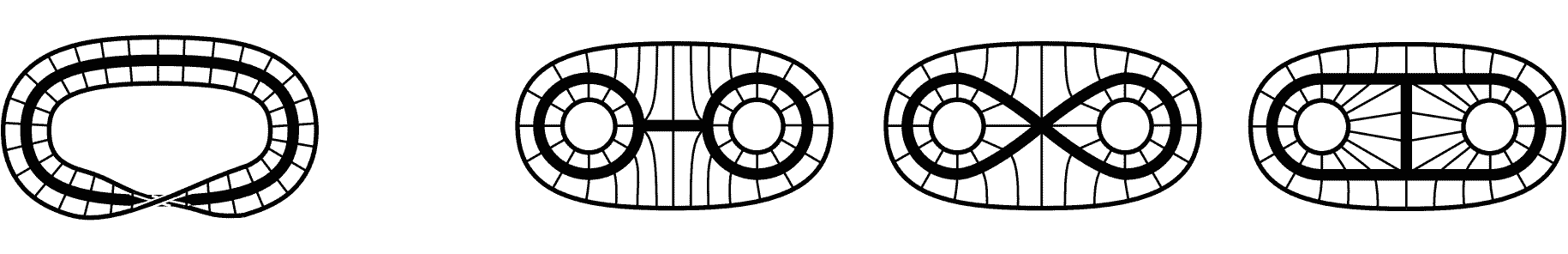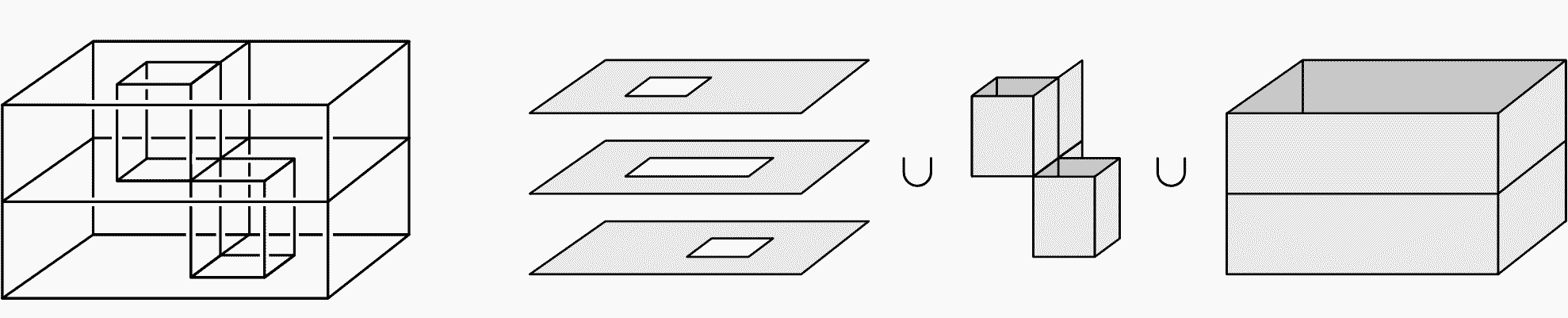## Van Kampen定理与同伦基本群的计算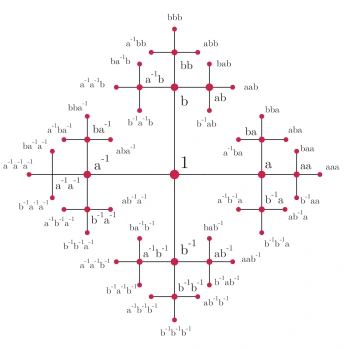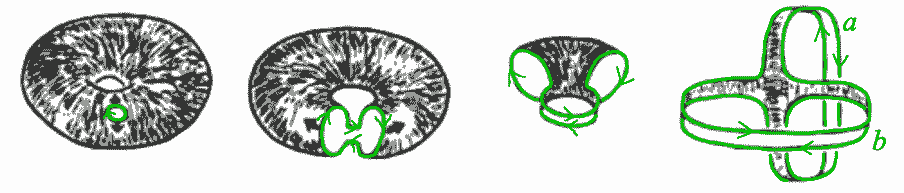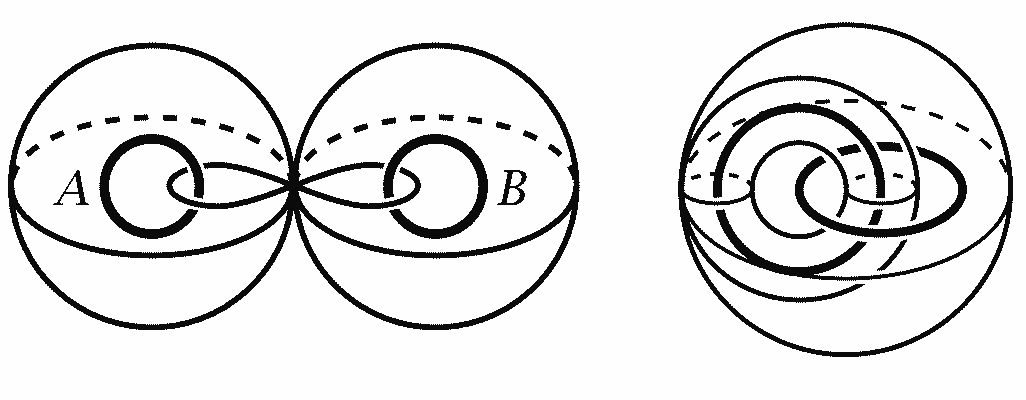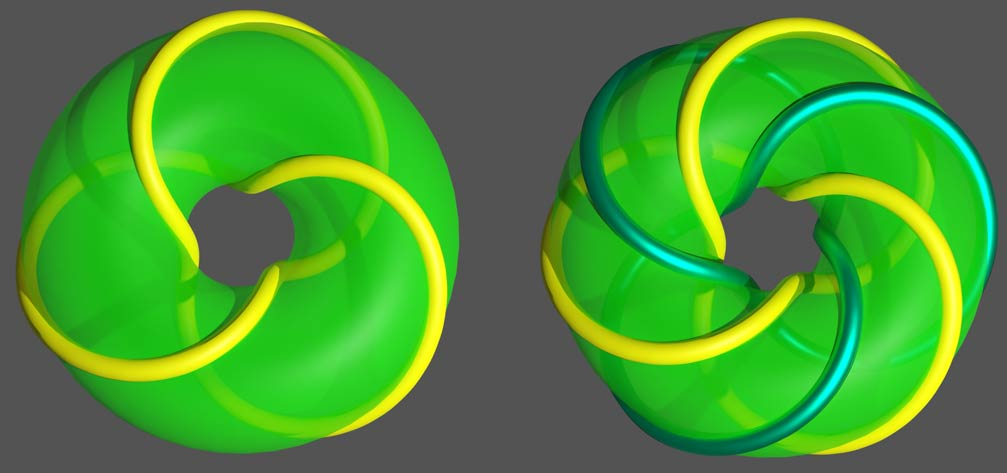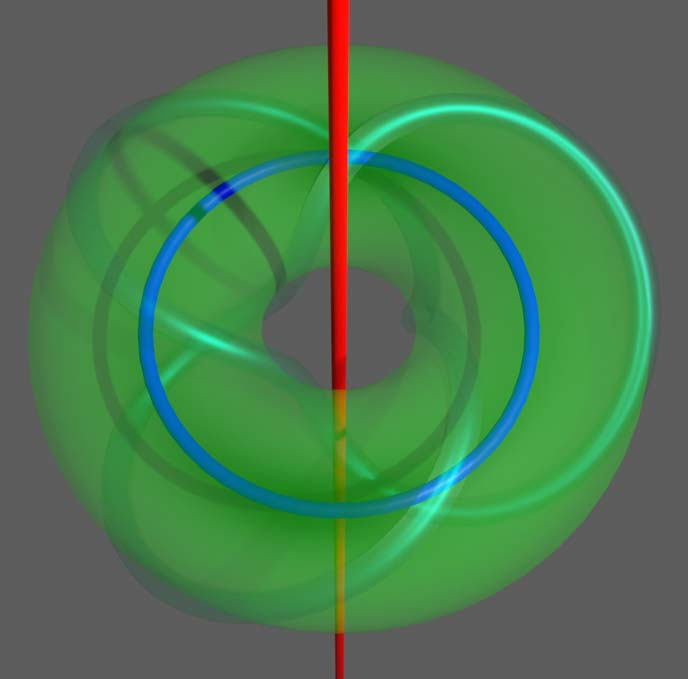## 覆盖空间与道路提升

1. 无限长的螺旋线延轴线投影得到圆周，这是圆周的一个无穷层的覆盖空间。
2. 观察下面这个很NB的gif动图中的球，它们跑出游戏边界就会从对面出现，这样的拓扑结构同胚于环面。因为上下左右都相连，我们可以把图片复制平铺满整个平面，图片中每件东西在这个平面中都有无数个，按照覆盖空间的定义，二维平面$\mathbb{R}^2$是环面$T^2$的一个无穷覆盖。其实这个动画只有30帧，循环播放，算上时间维，整个时空的拓扑结构是三维环面$T^3$，它是三个圆周的直积（同胚于各种圆环环曲胞），基本群是$\mathbb{Z}\times\mathbb{Z}\times\mathbb{Z}$。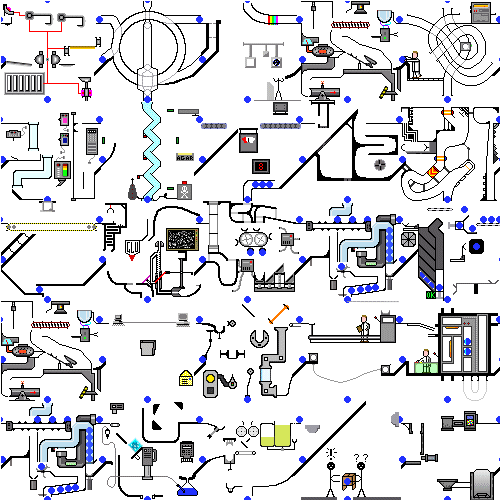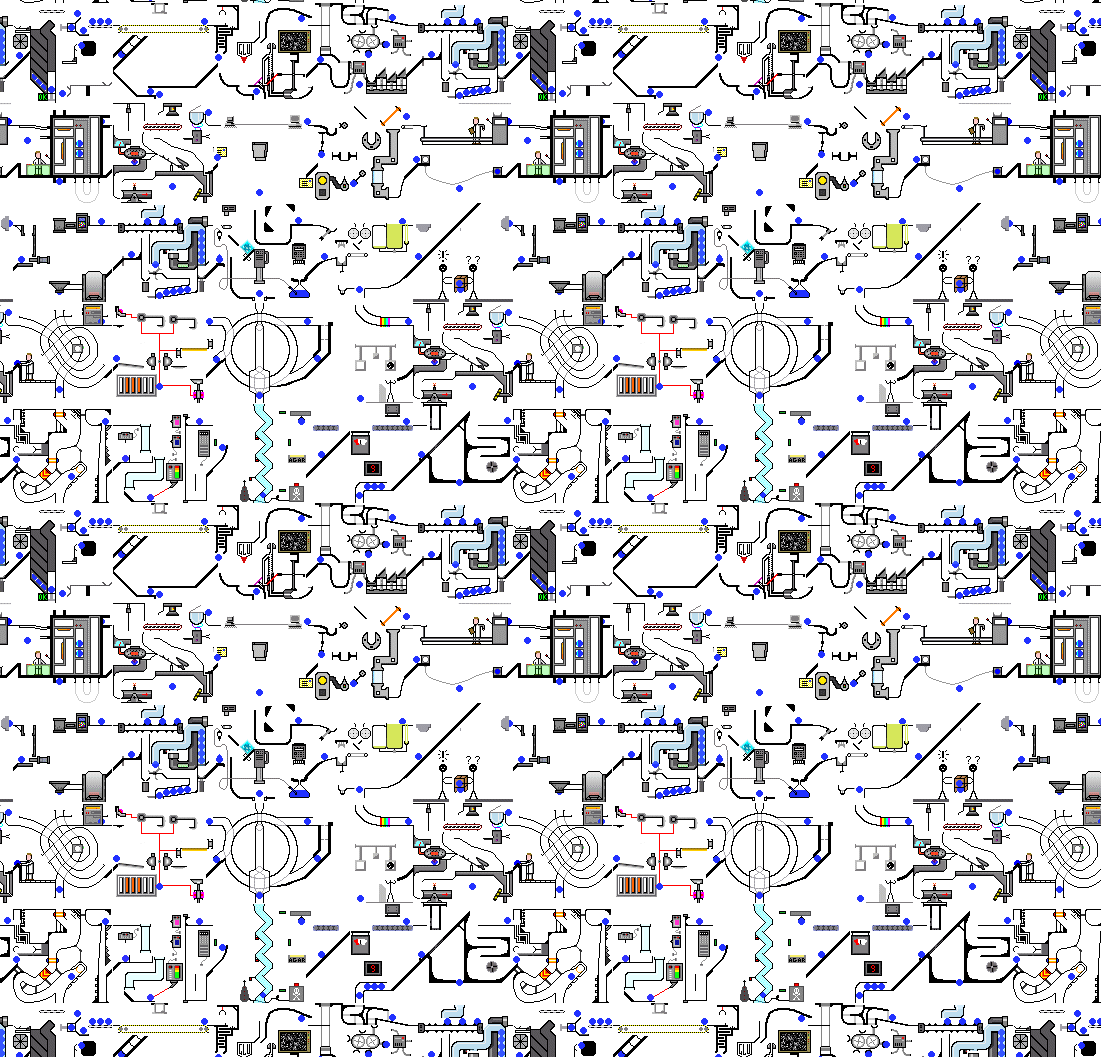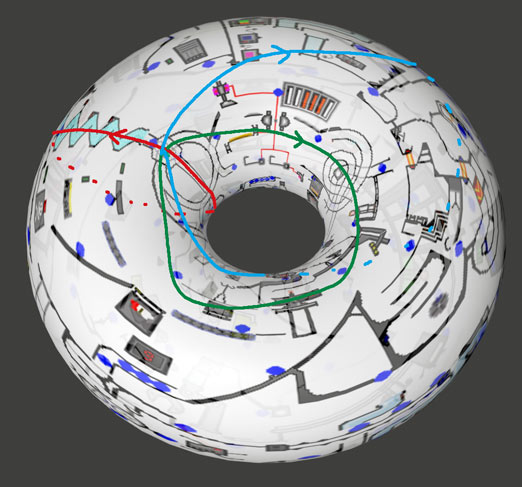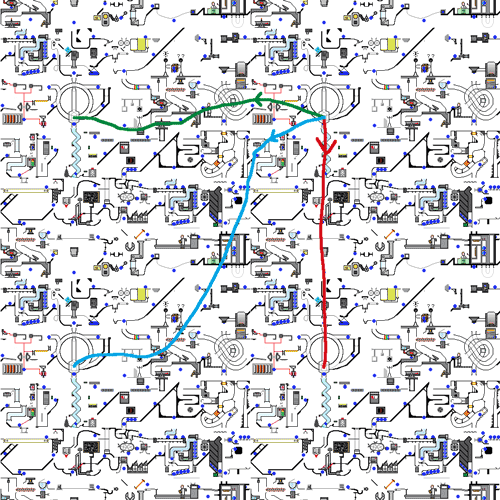### 覆盖空间分类与万有覆盖空间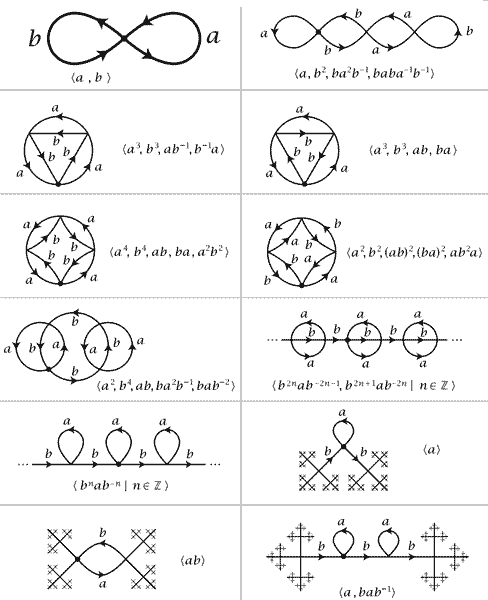## 高阶同伦群简介